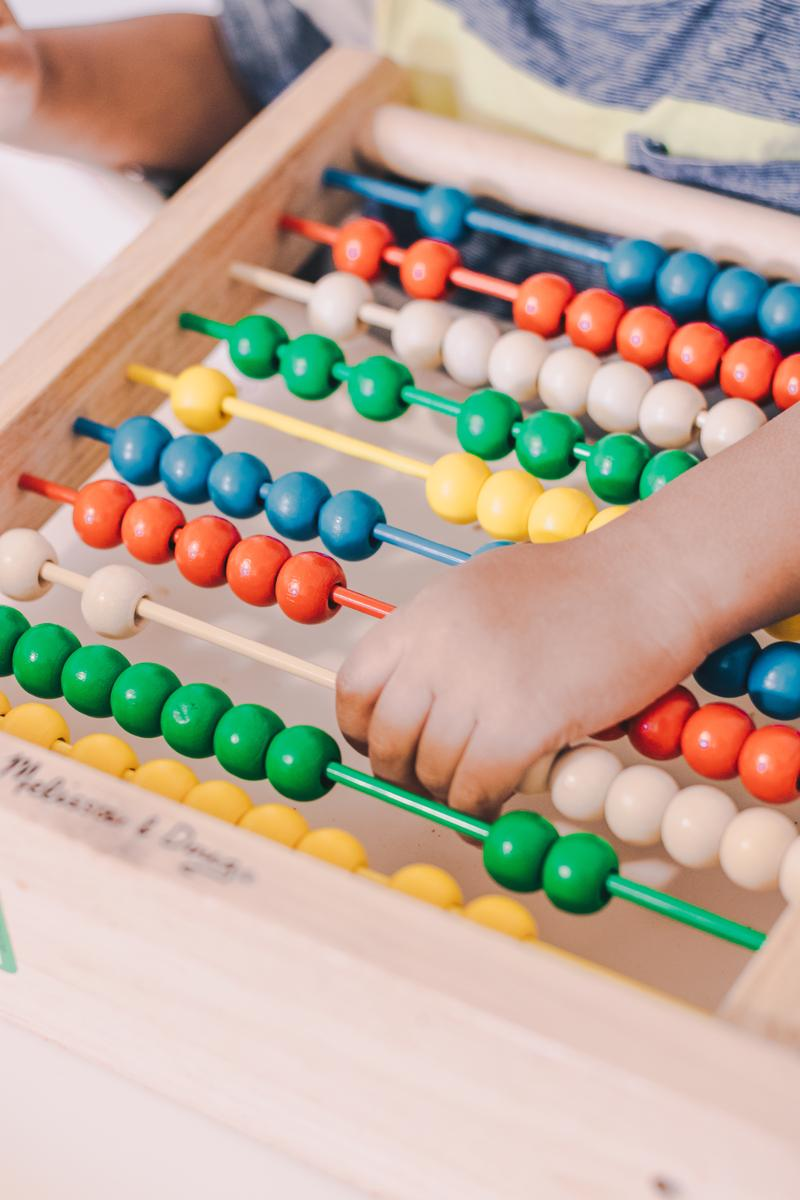Numeracy

Year Six

Dear Parents and Carers,

Over the past weeks I have outlined what the teaching and learning Mathematics curriculum encompasses for each year level. In this article I will focus on the final year, Year Six. In the classroom children may be working at level, below level or above level. Teachers differentiate the teaching and tasks to cater for the learning each child needs to access.

In Level 6, students work with prime, composite, square and triangular numbers and carry out mental, written and technology based computation to solve whole number problems involving all four operations. There are three major areas Number and Algebra, Measurement and Geometry, Statistics and Probability.

Number and Algebra

Number and place value

• Identify and describe properties of prime, composite, square and triangular numbers
• Select and apply efficient mental and written strategies and appropriate digital technologies to solve problems involving all four operations with whole numbers and make estimates for these computations
• Investigate everyday situations that use integers. Locate and represent these numbers on a number line

Money and financial mathematics

• Investigate and calculate percentage discounts of 10%, 25% and 50% on sale items, with and without digital technologies

Fractions and Decimals

• Compare fractions with related denominators and locate and represent them on a number line
• Solve problems involving addition and subtraction of fractions with the same or related denominators
• Find a simple fraction of a quantity where the result is a whole number, with and without digital technologies
• Add and subtract decimals, with and without digital technologies, and use estimation and rounding to check the reasonableness of answers
• Multiply decimals by whole numbers and perform divisions by non-zero whole numbers where the results are terminating decimals, with and without digital technologies
• Multiply and divide decimals by powers of 10 Multiply and divide decimals by powers of 10
• Make connections between equivalent fractions, decimals and percentages

Patterns and algebra

• Continue and create sequences involving whole numbers, fractions and decimals. Describe the rule used to create the sequence
• Explore the use of brackets and order of operations to write number sentences
• Design algorithms involving branching and iteration to solve specific classes of mathematical problems

Measurement and Geometry

Using units of measurement

• Connect decimal representations to the metric system
• Convert between common metric units of length, mass and capacity
• Solve problems involving the comparison
• Connect volume and capacity and their units of measurement
• Interpret and use timetables
• Measure, calculate and compare elapsed time

Shape

• Construct simple prisms and pyramids

Location and transformation

• Investigate the effect of combinations of transformations on simple and composite shapes, including creating tessellations, with and without the use of digital technologies
• Introduce the Cartesian coordinate system using all four quadrants

Geometric reasoning

• Investigate, with and without digital technologies, angles on a straight line, angles at a point and vertically opposite angles. Use results to find unknown angles

Statistics and Probability

Chance

• Describe probabilities using fractions, decimals and percentages.
• Conduct chance experiments with both small and large numbers of trials using appropriate digital technologies.
• Compare observed frequencies across experiments with expected frequencies

Data representation and interpretation

• Construct, interpret and compare a range of data displays, including side-by-side column graphs for two categorical variables.
• Interpret secondary data presented in digital media and elsewhere.
• Pose and refine questions to collect categorical or numerical data by observation or survey.

Kind regards,

Jennifer O’Connor

Assistant Principal